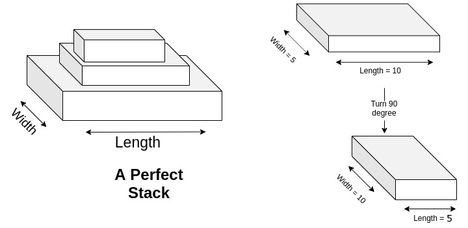# Practice on Toph

Participate in exhilarating programming contests, solve unique algorithm and data structure challenges and be a part of an awesome community.

# Messy Table

By TarikHassan · Limits 1s, 512 MB

Akib is a very good student but his reading table is very messy. One Friday afternoon, Akib is sitting idle and relaxing. To make his leisure time effective, he decides to clean his table.

First, Akib wants to sort his books and make a stack of them. He calls a stack of books perfect stack if a book's length and width is strictly less than the previous book.

For better explanation let's consider a stack of books A = {b1, b2, b3, ......, bm}. A is said to be a perfect stack if length and width of i-th book is strictly less than the length and width of the (i-1)-th book. The size of a perfect stack is the total number of books in a perfect stack.

Akib has n books. He also knows the value of two sides of each book (i.e. width and height of each book). In the process of making a perfect stack it is allowed to rotate a book 90 degrees. If you rotate a book 90 degrees then it's width becomes length and length becomes width.Now Akib wants to make a perfect stack of books, the size of which is as big as possible from the n books he has. Help Akib to solve this task.

## Input

The first line contains an integer n (1  ≤ n ≤ 5000) — the number of books Akib has. Then there will be n lines, each of them contains two integer numbers, Ai and Bi — the length of two sides of the i-th book (1 ≤ Ai,  Bi ≤ 100).

## Output

Print an integer which is the maximum perfect stack size.

## Samples

InputOutput
4
7 7
10 13
14 9
8 13
3

It is possible to make a perfect stack of maximum size 3. First Akib choose the 3rd book, then 4th book then the 1st book.
Hence the perfect stack A = {3, 4, 1} of size = 3.

InputOutput
2
15 15
15 15
1

Akib can make a perfect stack of a maximum size of 1 by choosing the 1st book or the 2nd book.

•DP

### Statistics

57% Solution Ratio

tanimahossainEarliest, Oct '19